RD Sharma Class 12 Solutions Chapter 7 Adjoint and Inverse of a Matrix Ex 7.1 are part of RD Sharma Class 12 Solutions. Here we have given RD Sharma Class 12 Solutions Chapter 7 Adjoint and Inverse of a Matrix Ex 7.1.

Here you can get free RD Sharma Solutions for Class 12 Maths of Chapter 7 Adjoint and Inverse of a Matrix Exercise 7.1. All RD Sharma Book Solutions are given here exercise wise for Adjoint and Inverse of a Matrix. RD Sharma Solutions are helpful in the preparation of several school level, graduate and undergraduate level competitive exams. Practicing questions from RD Sharma Mathematics Solutions for Class 12 Chapter 7 Adjoint and Inverse of a Matrix is proven to enhance your math skills.

 Class: 12th Class Chapter: Chapter 7 Name: Adjoint and Inverse of a Matrix Exercise: Exercise 7.1

## RD Sharma Class 12 Solutions Chapter 7 Adjoint and Inverse of a Matrix Ex 7.1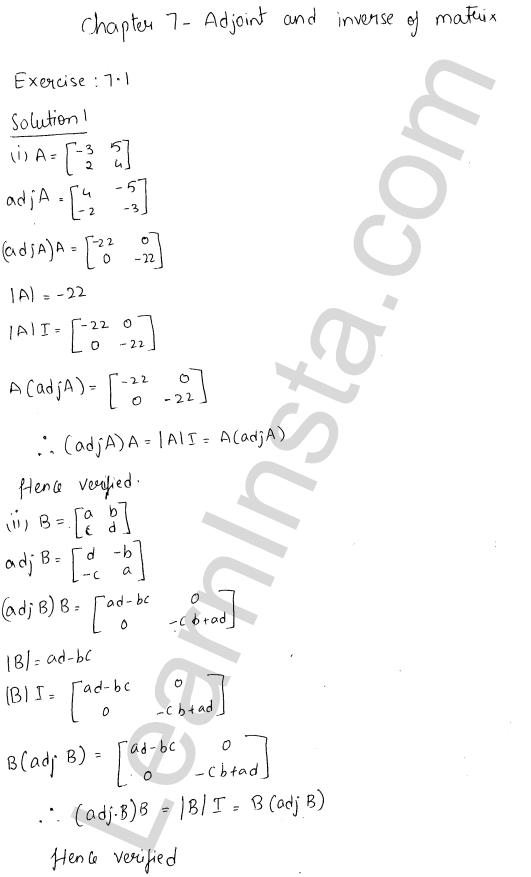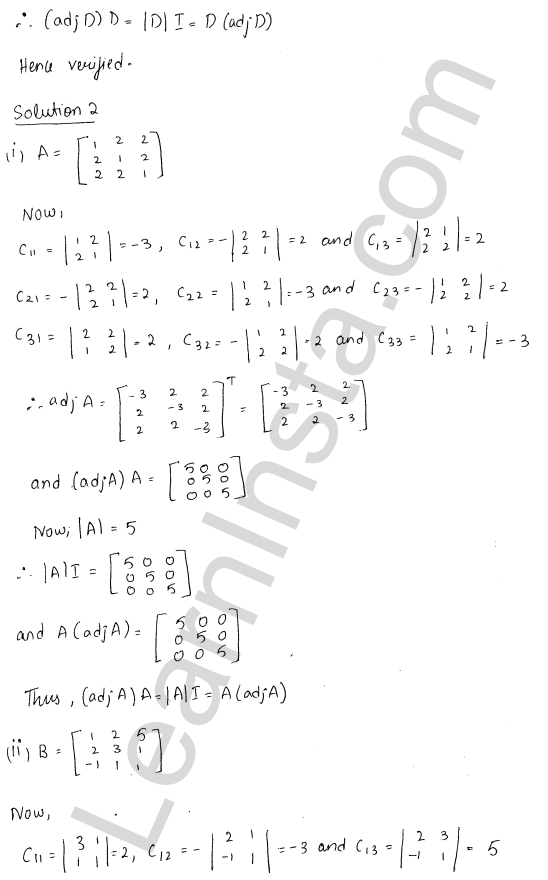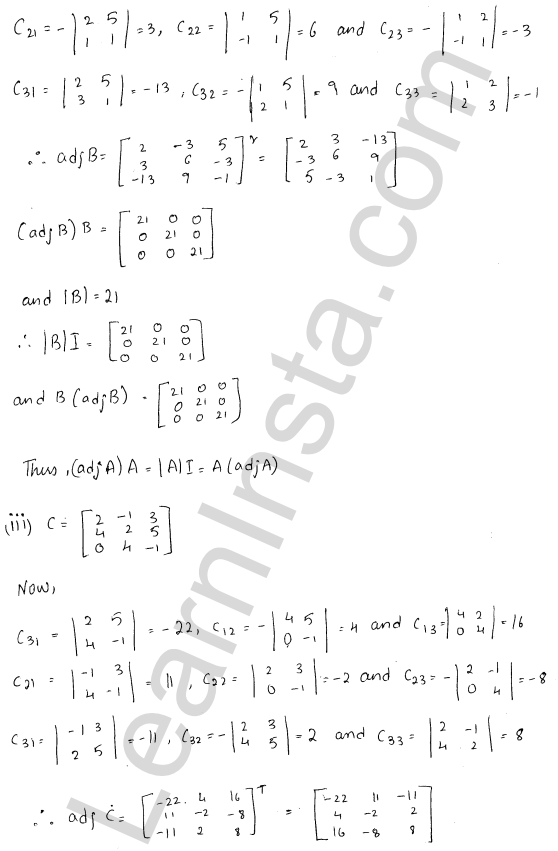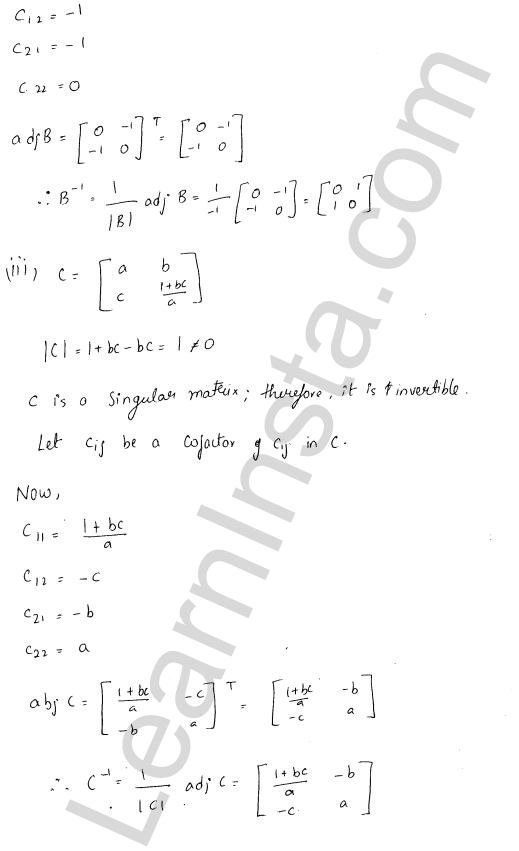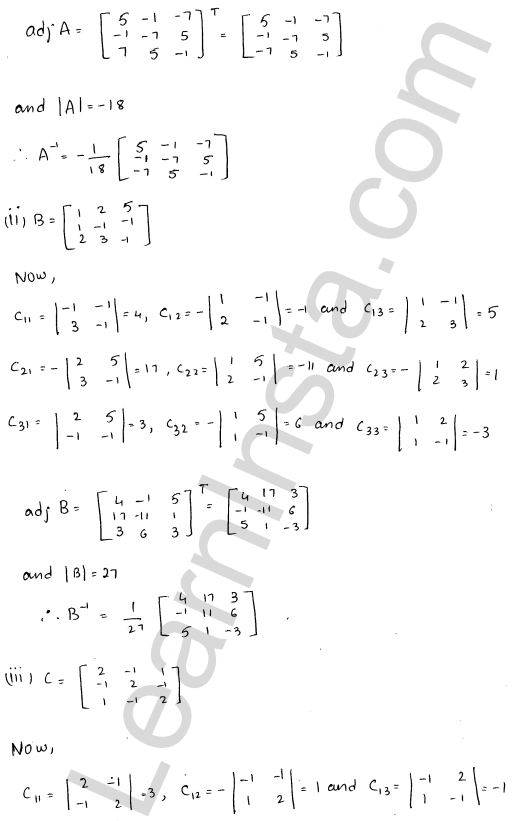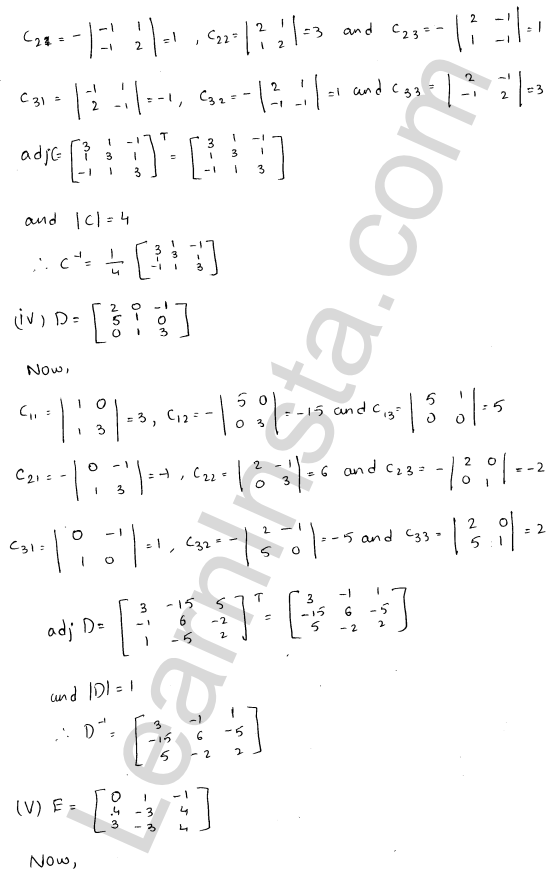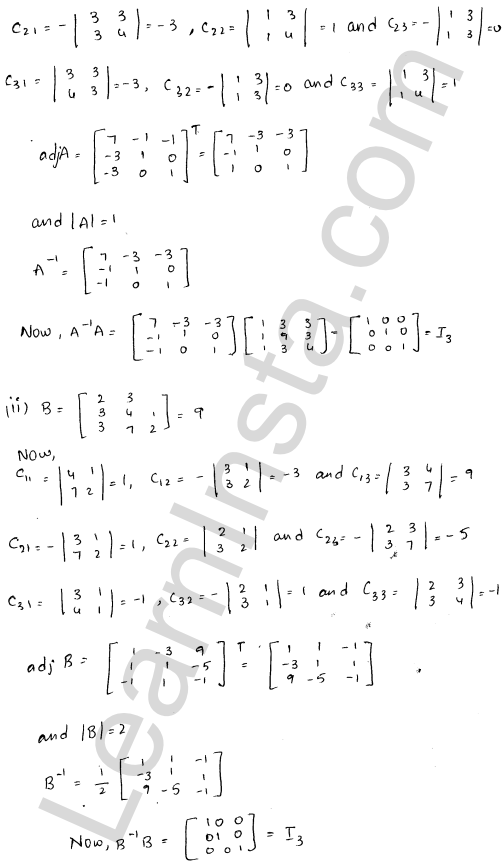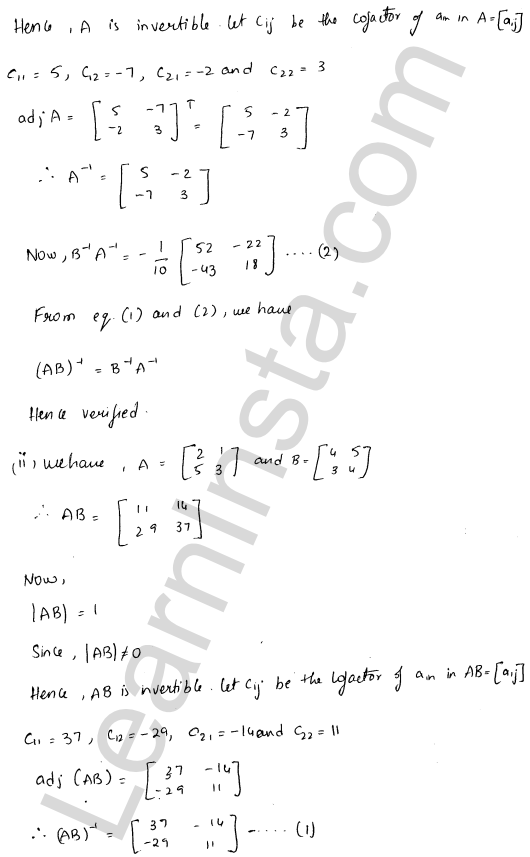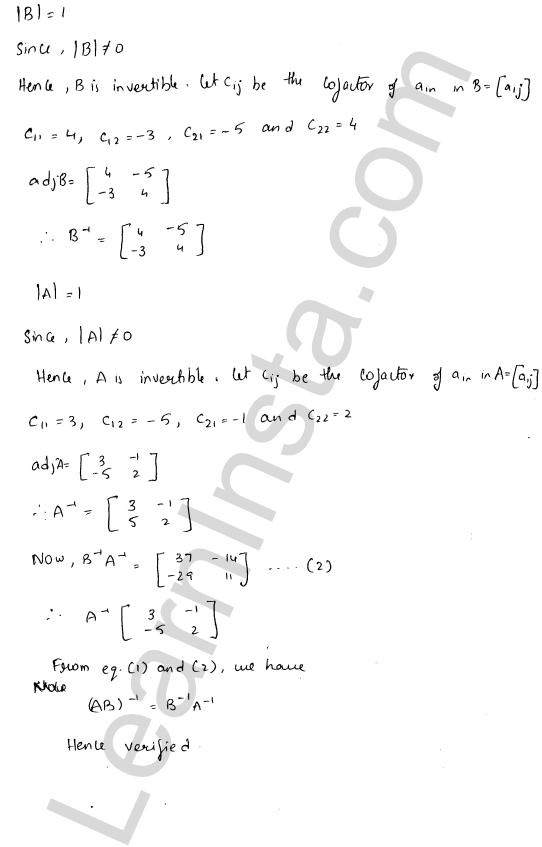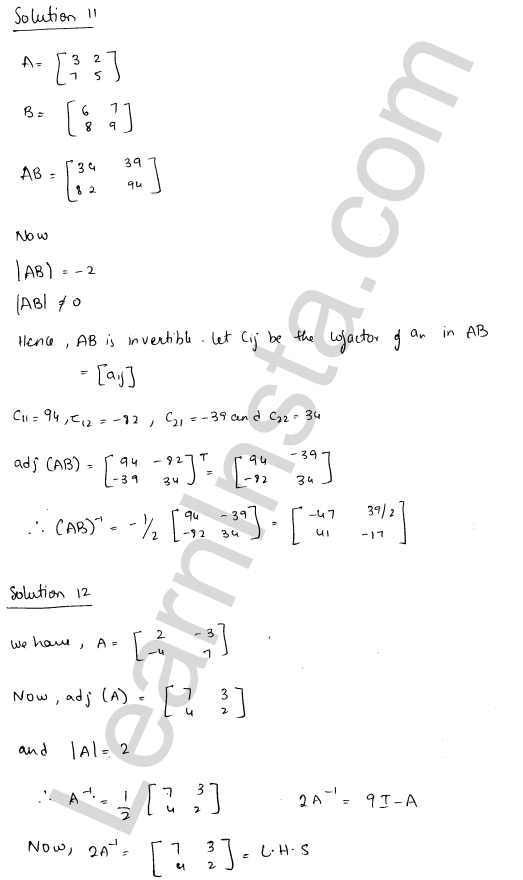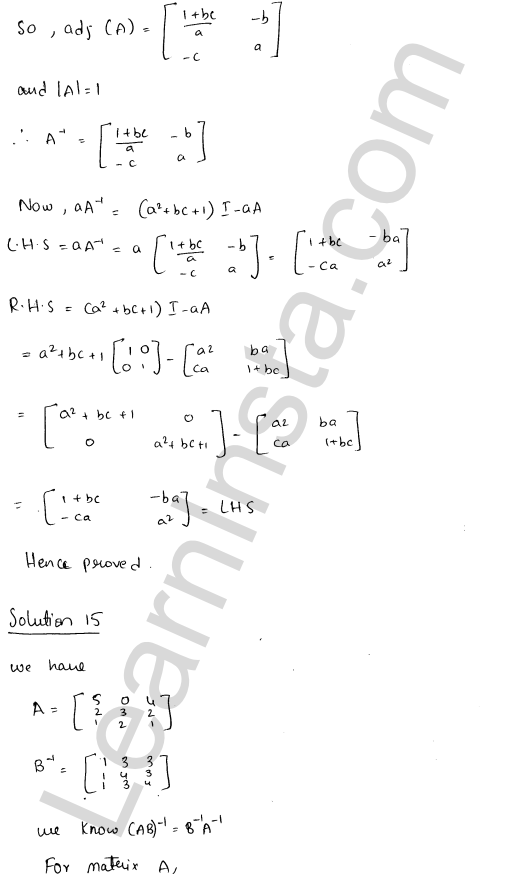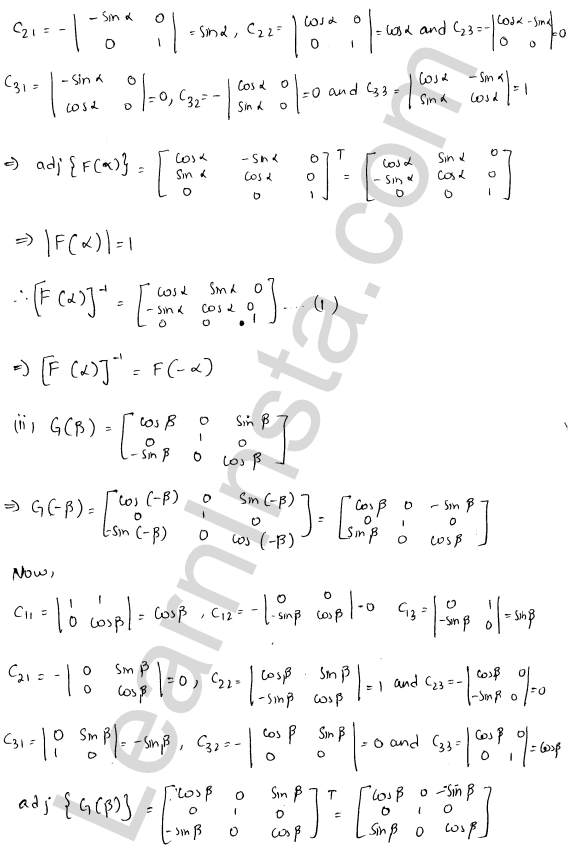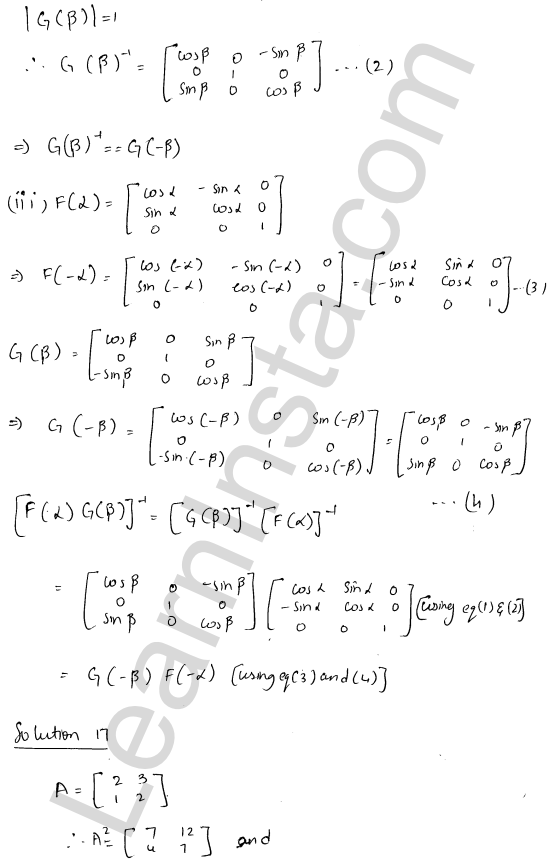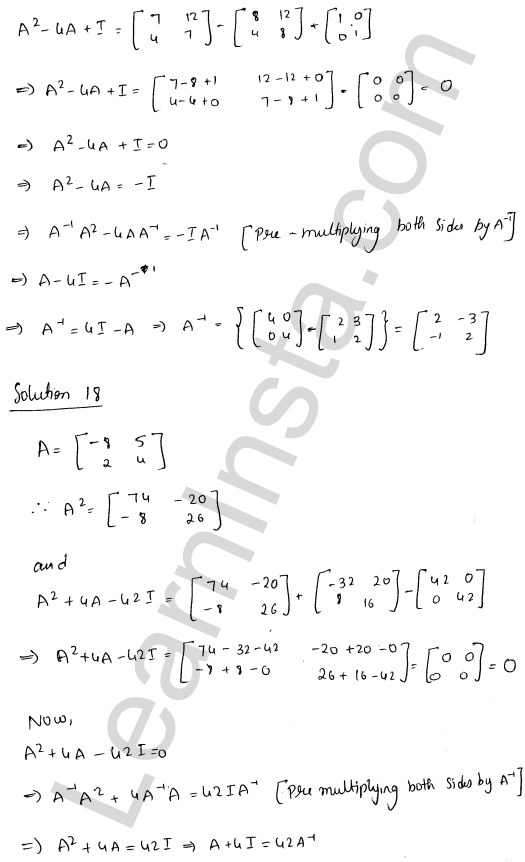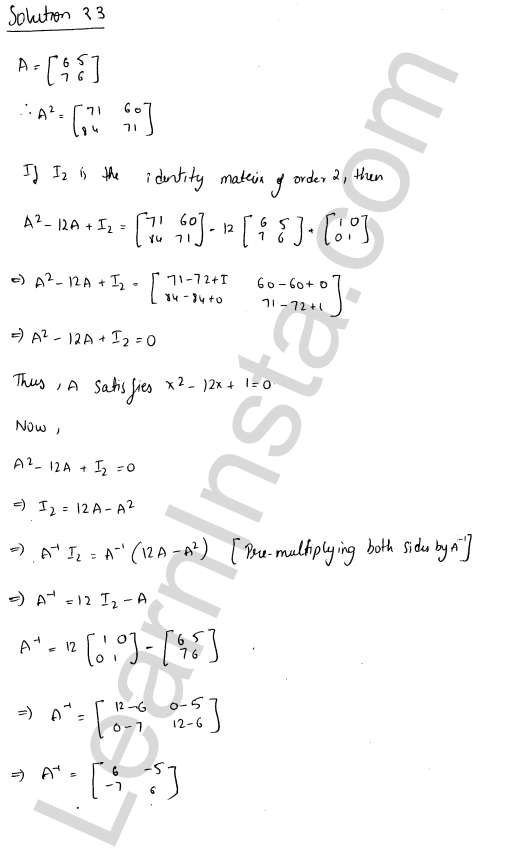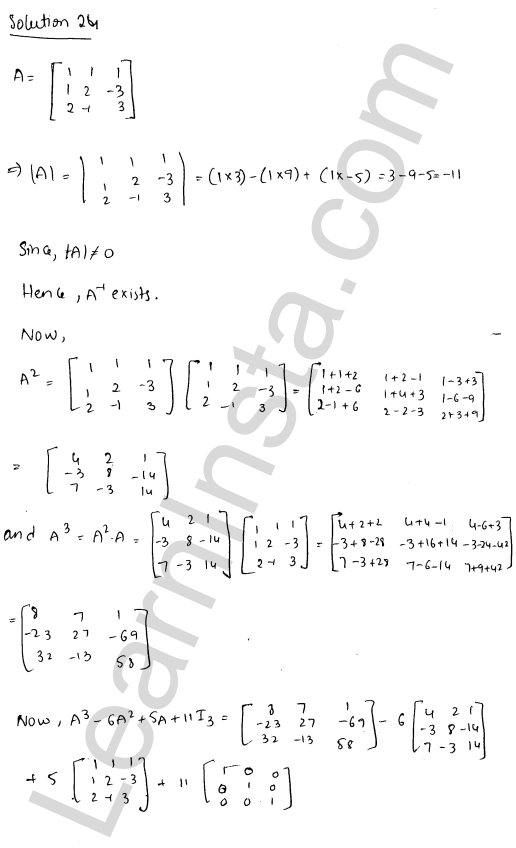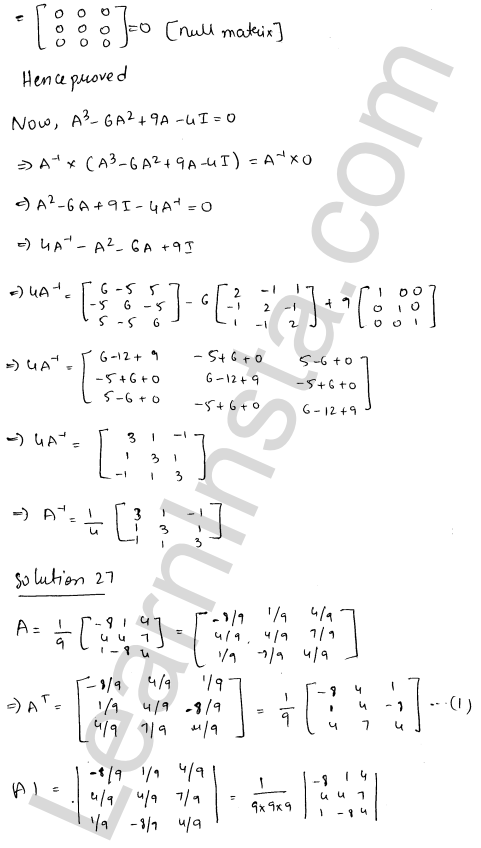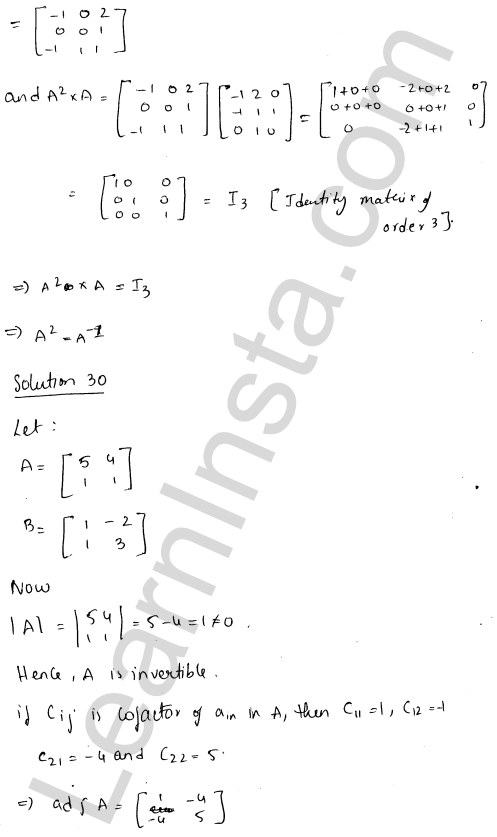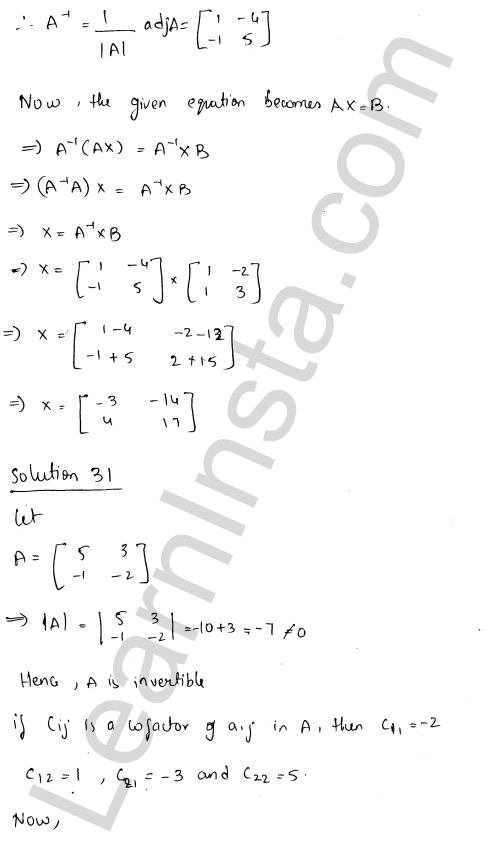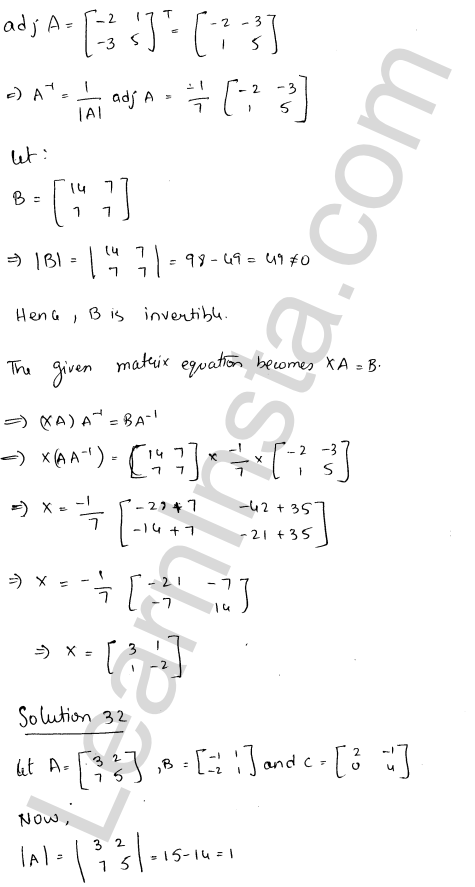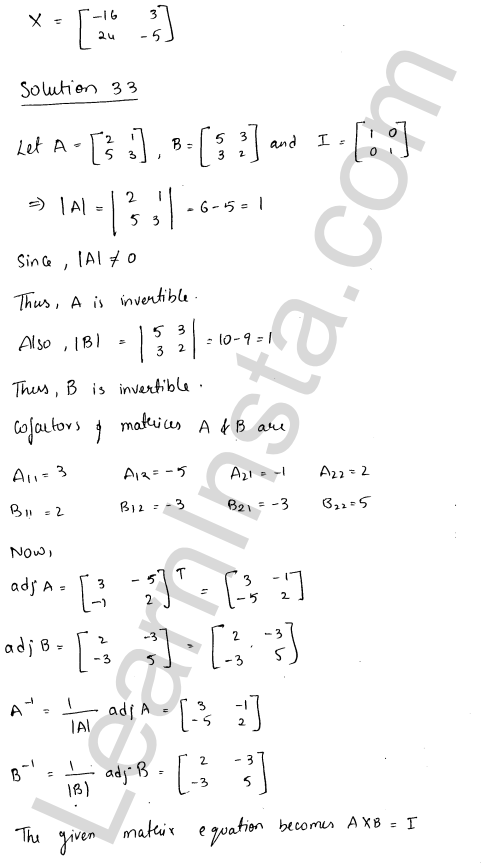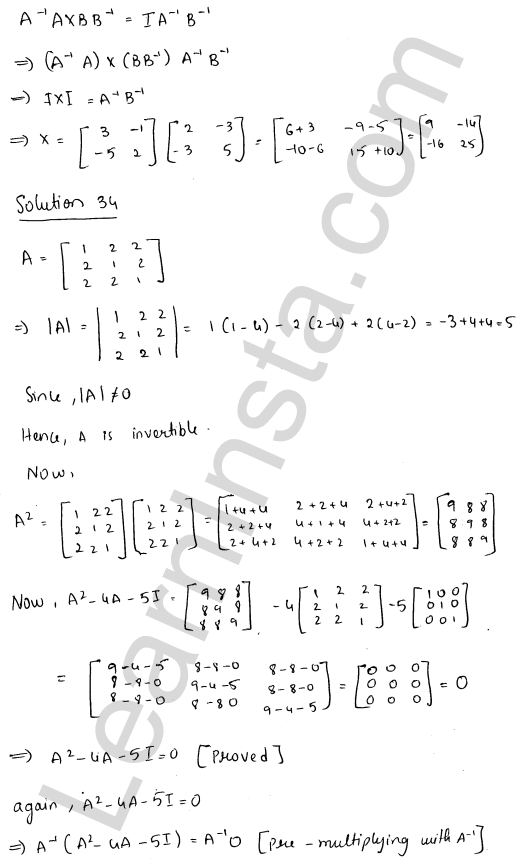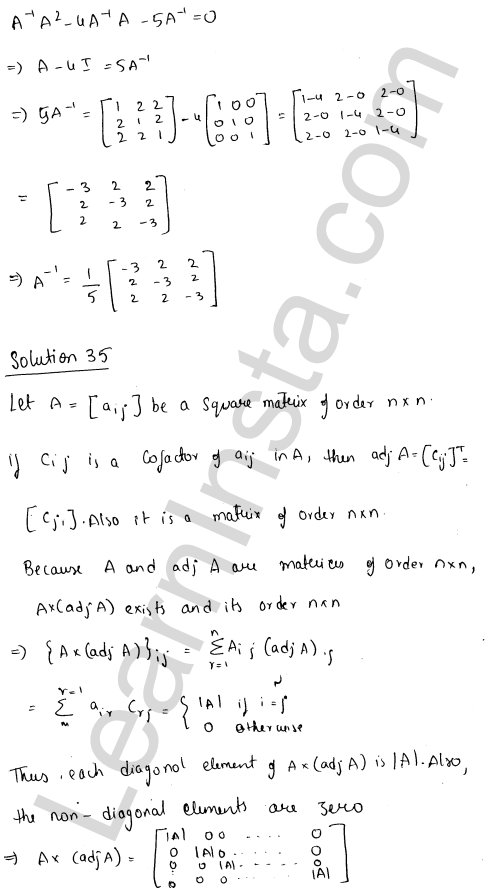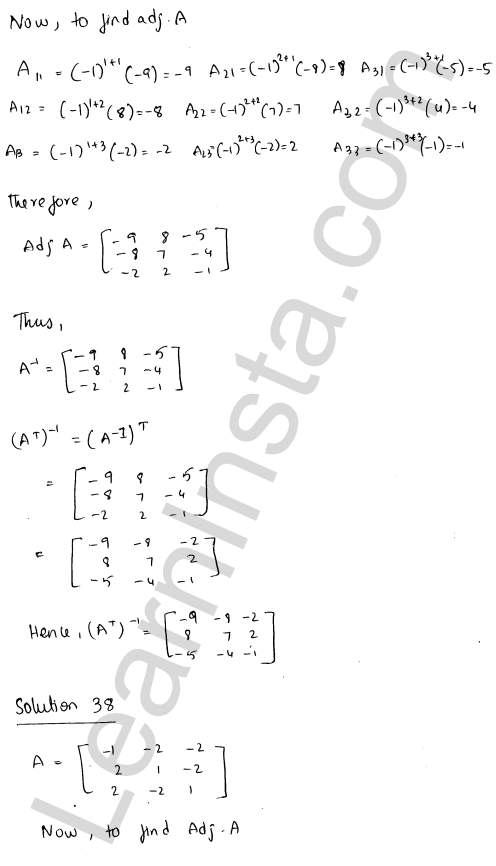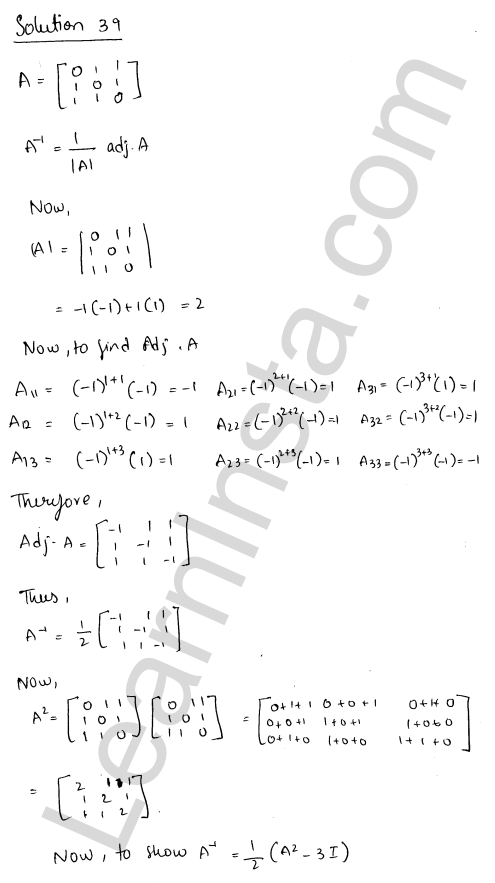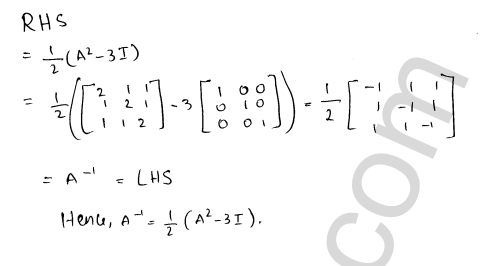We hope the RD Sharma Class 12 Solutions Chapter 7 Adjoint and Inverse of a Matrix Ex 7.1, help you. If you have any query regarding RD Sharma Class 12 Solutions Chapter 7 Adjoint and Inverse of a Matrix Ex 7.1, drop a comment below and we will get back to you at the earliest.